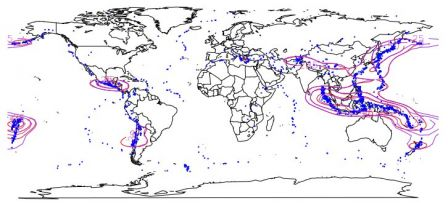Want to share your content on R-bloggers? click here if you have a blog, or here if you don't.

I few years ago, while I was working on kernel based density estimation on compact support distribution (like copulas) I went through a series of papers on circular distributions. By that time, I thought it was something for mathematicians working on weird spaces…. but during the past weeks, I saw several potential applications of those estimators.
• circular data density estimation
Consider the density of an angle say, i.e. a function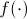such thatwith a circular relationship, i.e.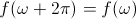. It can be seen as an invariance by rotation.
von Mises proposed a parametric model in 1918 (see here or there), assuming that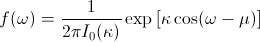whereis Bessel modified function of order 1,(which is simply a normalization parameter). There are two parameters here,(some concentration parameter) and mu a direction.
From a series of observed angles, the maximum likelihood estimator for kappa is solution of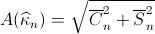whereandand where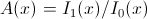, where those functions are modified Bessel functions. Well, that estimator is biased, but it is possible to improve it (see here or there). This can be done easily in R (actually Jeff Gill – here – used that package in several applications). But I am not a big fan of that technique….
• density estimation for hours on simulated data
A nice application can be on the estimation of the daily density of a temporal events (e.g. phone calls as we’ll see later on, or email arrival time). Letis the time (in hours) for theth observation (theth phone call received). Then set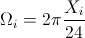The time is now seen as an angle. It is possible to consider the equivalent of an histogram,
```set.seed(1)
library(circular)
X=rbeta(100,shape1=2,shape2=4)*24
Omega=2*pi*X/24
Omegat=2*pi*trunc(X)/24
plot(Ht, stack=FALSE, shrink=1.3, cex=1.03,
points(Ht, rotation = "clock", zero =c(rad(90)),
col = "1", cex=1.03, stack=TRUE )

rose.diag(Ht-pi/2,bins=24,shrink=0.33,xlim=c(-2,2),ylim=c(-2,2),
axes=FALSE,prop=1.5)```or a kernel based estimation of the density (the gray line on the right).
```circ.dens = density(Ht+3*pi/2,bw=20)
plot(Ht, stack=TRUE, shrink=.35, cex=0, sep=0.0,
axes=FALSE,tol=.8,zero=c(0),bins=24,
xlim=c(-2,2),ylim=c(-2,2), ticks=TRUE, tcl=.075)
lines(circ.dens, col="darkgrey", lwd=3)
text(0,0.8,"24", cex=2); text(0,-0.8,"12",cex=2);
text(0.8,0,"6",cex=2); text(-0.8,0,"18",cex=2)```
The c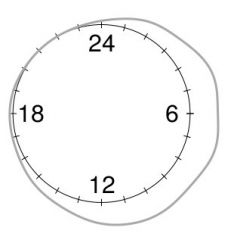ode looks rather simple. But I am not very comfortable using codes that I do not completely understand. So I did my own. The first step was to get a graph similar to the one we have on the right, except that I prefer my own kernel based estimator. The idea is that instead of estimating the density on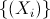, we estimate it on the sample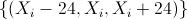. Then we multiply by 3 to get the density only on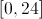. For the bandwidth, I took the same as the one that we would have taken onThe code is simply the following

```U=seq(0,1,by=1/250)
O=U*2*pi
U12=seq(0,1,by=1/24)
O12=U12*2*pi
X=rbeta(100,shape1=2,shape2=4)*24
OM=2*pi*X/24
XL=c(X-24,X,X+24)
d=density(X)
d=density(XL,bw=d\$bw,n=1500)
I=which((d\$x>=6)&(d\$x<=30))
Od=d\$x[I]/24*2*pi-pi/2
Dd=d\$y[I]/max(d\$y)+1

plot(cos(O),-sin(O),xlim=c(-2,2),ylim=c(-2,2),
type="l",axes=FALSE,xlab="",ylab="")
for(i in pi/12*(0:12)){
abline(a=0,b=tan(i),lty=1,col="light yellow")}
segments(.9*cos(O12),.9*sin(O12),1.1*cos(O12),1.1*sin(O12))
lines(Dd*cos(Od),-Dd*sin(Od),col="red",lwd=1.5)
text(.7,0,"6"); text(-.7,0,"18")
text(0,-.7,"12"); text(0,.7,"24")
R=1/24/max(d\$y)/3+1
lines(R*cos(O),R*sin(O),lty=2)```

Note that it is possible to stress more (visually) on hours having few phone calls, or a lot (compared with an homogeneous Poisson process), e.g.

```plot(cos(O),-sin(O),xlim=c(-2,2),ylim=c(-2,2),
type="l",axes=FALSE,xlab="",ylab="")
for(i in pi/12*(0:12)){
abline(a=0,b=tan(i),lty=1,col="light yellow")}
segments(2*cos(O12),2*sin(O12),1.1*cos(O12),1.1*sin(O12),
col="light grey")
segments(.9*cos(O12),.9*sin(O12),1.1*cos(O12),1.1*sin(O12))
text(.7,0,"6")
text(-.7,0,"18")
text(0,-.7,"12")
text(0,.7,"24")
R=1/24/max(d\$y)/3+1
lines(R*cos(O),R*sin(O),lty=2)
AX=R*cos(Od);AY=-R*sin(Od)
BX=Dd*cos(Od);BY=-Dd*sin(Od)
COUL=rep("blue",length(AX))
COUL[R<Dd]="red"
CM=cm.colors(200)
a=trunc(100*Dd/R)
COUL=CM[a]
segments(AX,AY,BX,BY,col=COUL,lwd=2)
lines(Dd*cos(Od),-Dd*sin(Od),lwd=2)```

We get here those two graphs,To be honest, I do not really like that representation - even if it looks nice. If we compare that circular representation to a more classical one (from 0:00 till 23:59 one the graph on the left, below), I do have a problem to interpret the areas in blue and pink.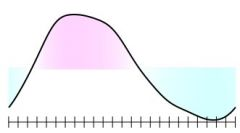On the left, we compare two densities, so the area in pink is the same as the area in blue. But here, it is no longer the case: the area in pink is always larger to the one in blue. So it might help so see when we have a difference, but there is a scaling issue that we cannot discuss further... But less us see if we can use that estimation technique to several problems.
• density of wind directionA standard application when studying angles is wind direction. For instance, in Montréal, it is possible to find hourly observations, starting in 1974 (we just need a R robot to pick up the information, but I'll tell more about that in another post, someday). Here, we have directly an angle. So we can use a code rather similar to the one used above to estimate the distribution of wind direction in Montréal.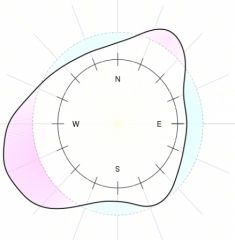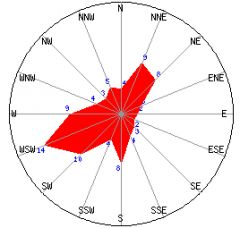Note that our estimate is consistent with several graphs that can be found on meteorological websites (e.g. the one above on the right, that was found here).
• density of 911 phone calls
In a recent post (here) I wanted to check about the "midnight crime" myth, using hours of 911 phone calls in Montréal.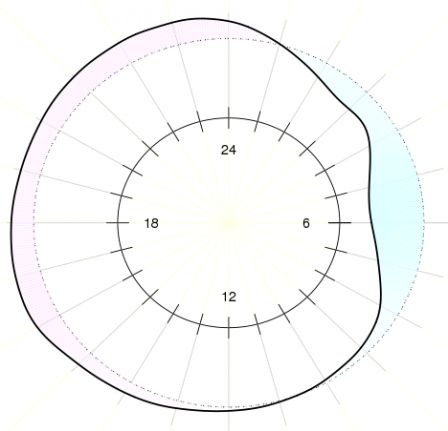That was for all phone calls. But if we look more specifically, for burglaries, we have the distribution on the left, and for conflicts the one on the right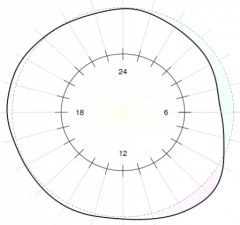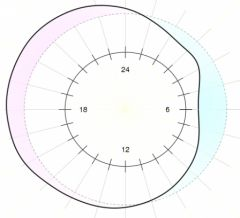while for gun shots, we have the distribution on the left, and for "troubles" (basically people making too much noisy in parties) or "noise" the one on the right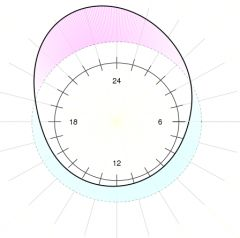We do clearly observe that gun shots occur a bit before midnight. See also here for another study, but this time in NYC (thanks @PAC for the link).
• density of earth temperatures, or earthquakes
Of course it is also possible to work in higher dimension. Before, we went from densities onto densities on the unit circle. But similarly, it is possible to go fromto the unit sphere. A nice application being global climate studies,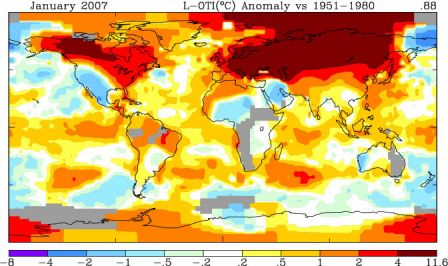The idea being that point on the left above are extremely close to the one on the right. An application can be e.g. on earthquakes occurrence. Data can be found here.
```library(ks)
X=cbind(EQ\$Longitude,EQ\$Latitude)
Hpi1 = Hpi(x = X)
DX=kde(x = X, H = Hpi1)
library(maps)
map("world")
points(X,cex=.2,col="blue")
Y=rbind(cbind(X[,1],X[,2]),cbind(X[,1]+360,X[,2]),
cbind(X[,1]-360,X[,2]),cbind(X[,1],X[,2]+180),
cbind(X[,1]+360,X[,2]+180),cbind(X[,1]-360,X[,2]+180),
cbind(X[,1],X[,2]-180),cbind(X[,1]+360,
X[,2]-180),cbind(X[,1]-360,X[,2]-180))
DY=kde(x = Y, H = Hpi1)
library(maps)